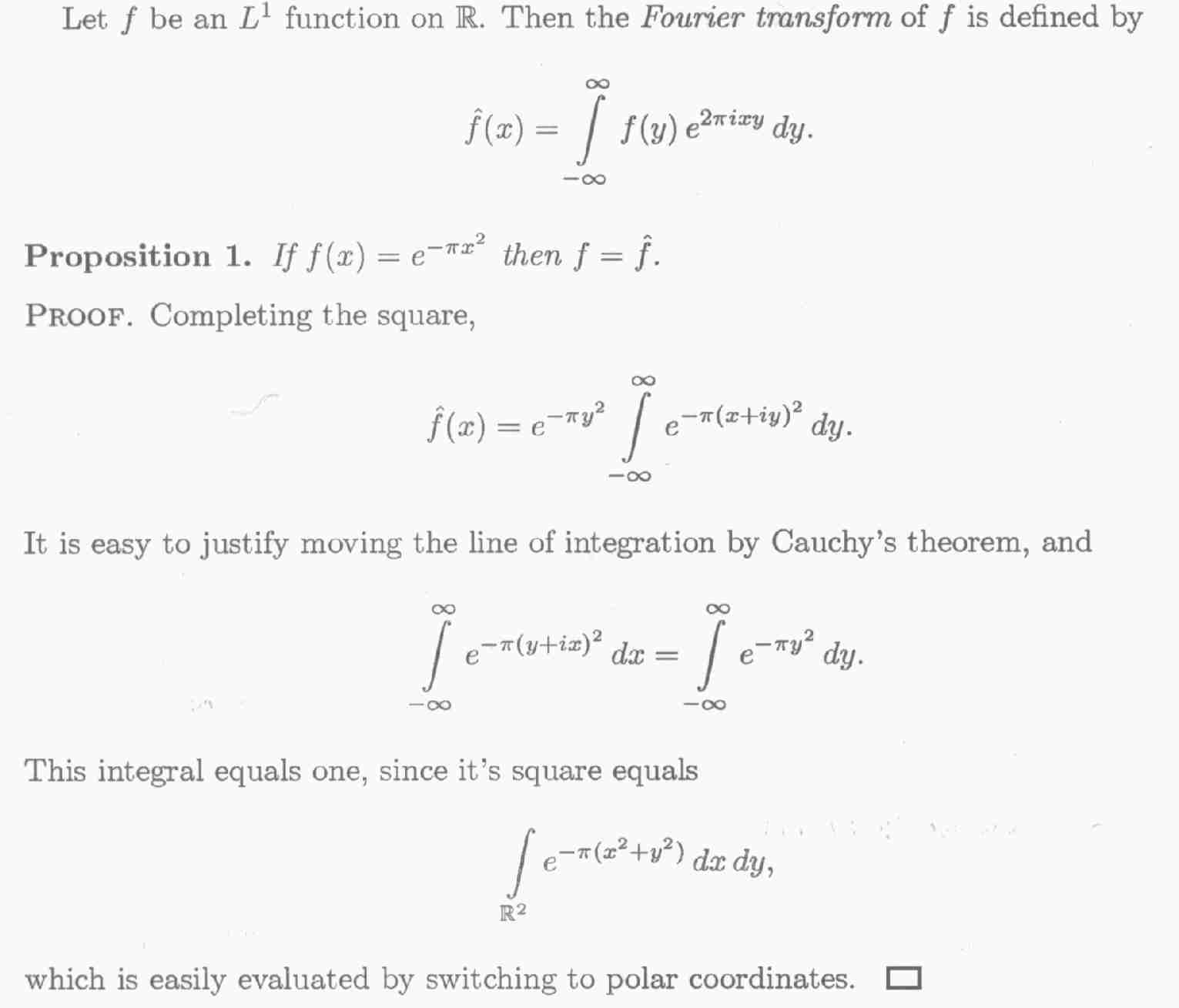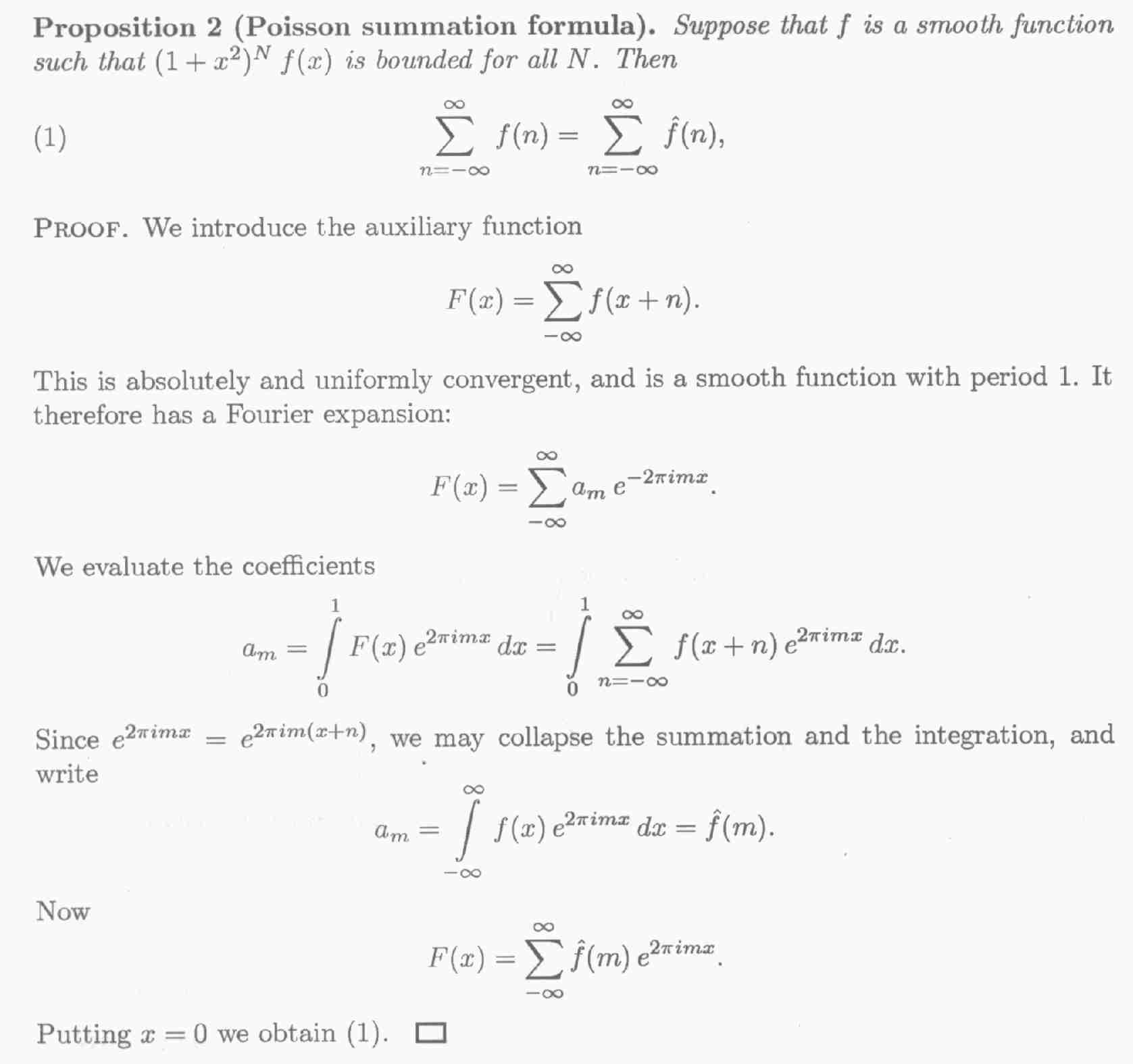### D. Bump's notes on the Poisson Summation Formula

[These are taken from p.1-2 of Bump's notes on the Riemann zeta function.]Although in the explanation above, the Poisson Summation Formula is presented as a straightforward result of Fourier analysis, it is possible to interpret it as a kind of simple "trace formula" on a torus. This is outlined in:

H.P. McKean, "Selberg's trace formula as applied to a compact Riemannian surface", Communications in Pure and Applied Mathematics 25 (1972) 225-246.

The PSF is seen in this context as relating the spectrum of the Laplacian on a torus to the lengths of its closed geodesics. This interpretation allows for generalisation, as the torus is a compact Riemann surface of genus 1. A general genus n compact Riemann surface also has a Laplacian spectrum and a set of shortest path lengths in each deformation class, and it turns out that these sets of values can be related according to an analogous formula, essentially the Selberg trace formula.

archive      tutorial      mystery      new      search      home      contact